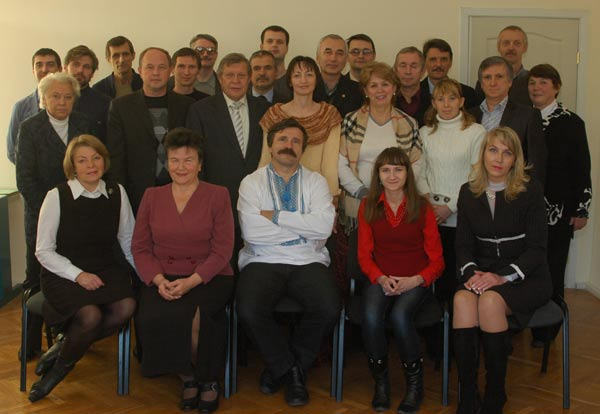### Department CM

Head — Doctor of Physical and Mathematical Sciences, Professor, Corresponding Member of NAS of Ukraine Lyashko S.I.The Department of CM prepares specialists in the field of numerical analysis, optimal control and mathematical modeling.
The department has a research laboratory of computational methods in the mechanics of continuous media. Head of the Laboratory - Candidate of Phys. Sciences, Senior Researcher Stellya O.B.
Mathematical analysis, functional analysis, equations of mathematical physics, theory of functions of complex variable, programming, numerical methods, optimization methods.

### Department of Computational Mathematics

22

#### Teachers

6 professors and doctors of science, 11 associate professors and candidates of sciences

26

#### Cources

The staff of the department read 26 normative and special courses

55

#### Years

The Department of Computational Mathematics was founded in December 1967

### Staff:

Full name: Office Research interests
Prof. Sergiy I. Lyashko 216 informatics, system simulation and optimization
Prof. Arkadii O. Chikrii 212 applied nonlinear analysis, theory of functions of a real variable, mathematical control theory, theory of dynamic games, theory of moving target search
Prof. Valerii K. Zadiraka 213 computation theory, mathematical simulation, digital signal processing, information security
Prof. Anatolii V. Gladkii 210 methods of mathematical simulation of steady (non-steady) problems of applied mathematics and ecology, numerical-analytical methods of simulation of wave processes described by elliptic (parabolic Schredinger–like) wave equations with complex not self-adjoint operator
Prof. Dmitry A. Nomirovskii 214 optimal control theory, partial differential equation, functional analysis
Prof. Bogdan V. Rublyov 236 numerical geometry, numerical topology
Prof. Oleksandr M. Khimich 212 Theory of perturbations and errors, numerical methods and computer algorithms, methods of parallel computations, software engineering, intelligent computer systems, mathematical simulation
Prof. Dmitry A. Klyushin 214 numerical mathematics, mathematical simulation, statistical pattern recognition, functional analysis
Prof. Vladimir V. Semenov 217 variational problems, theory of optimal control, partial differential equation, functional analysis, numerical algorithms
Assoc. Prof. Gennadii V. Sandrakov 212 asymptotic methods, theory of averaging, equations of mathematical physics, mathematical hydrodynamics, variational problems, numerical mathematics, simulation, functional analysis
Assoc. Prof. Yurii V. Goncharenko 209 mathematical analysis
Assoc. Prof. Larisa T. Adjubey 209 theory of optimal control, mathematical methods in economics
Assoc. Prof. Irina M. Aleksandrovich 210 integral representation of solutions of Helmholtz equation and integral axe-symmetric Helmholtz equation, differential and integral operators defining solutions of equations and systems of elliptic and hyperbolic types
Assoc. Prof. Vasyl I. Garkusha 213 variational problems, optimal control theory, partial differential equations, problems of applied aerohydrodynamics, algorithms of control and filtration
Assoc. Prof. Olena F. Kashpur 213 approximation of nonlinear operators, spectral theory of operators, partial differential equations
Assoc. Prof. Anatolii V. Kuzmin 213 partial differential equations, numerical methods in hydrodynamics, mathematical methods of analysis of remote sensing of Earth, mathematical methods of analysis of modern land policy in Ukraine
Assoc. Prof. Oleksander I. Molodtsov 208 mathematical analysis, numerical methods in mathematical physics
Assoc. Prof. Andriy I. Ryzhenko 213 theory of numerical methods, numerical methods for solving problems of mathematical physics, numerical methods for solving problems of heat and mass transport
Assoc. Prof. Dmitry I. Chernii 407 partial differential equations, numerical methods for singular integral equations, numerical technology for vortical flows and evolution processes
Assoc. Prof. Andriy V. Anikushin 215 linear operator equations, differential and integrodifferential partial differential equations, functional analysis
Assist. Prof. Viacheslav V. Onotsky 237 development of algorithms and software for solving Navier-Stocks systems, parabolic and hyperbolic equations, finite-difference algorithms, problems of identification and optimal control, ill-conditioned systems of linear algebraic equations
Assist. Prof. Katerina M. Golubeva 215 computational mathematics, mathematical simulation, statistical pattern recognition
Assist. Prof. Andriy L. Hulianytskyi 215 computational mathematics, mathematical modeling, statistical theory of pattern recognition
Assist. Prof. Sergiy V. Denisov 215 optimization methods